### Site swap laboratory

Laboratory fifteen in physical science sought to push the boundaries on the mathematical box for the students. In laboratory one a quote from Freeman Dyson was used to start a journey through the mathematical models that explain physical systems. Dyson calculated how an electron ought to behave. Later someone went into a laboratory and the electron behaved as predicted by the mathematical model.In laboratory two a linear model predicted the location of a rolling ball. In laboratory three a falling ball obeyed a quadratic mathematical relationship. The behavior of a marble rolling off of a banana leaf obeyed a square root relationship. And in laboratory four the marbles knew what to do in order to mathematically conserve momentum. Sound, the relative depth of an image, and Ohm's law all exhibited linear relationships.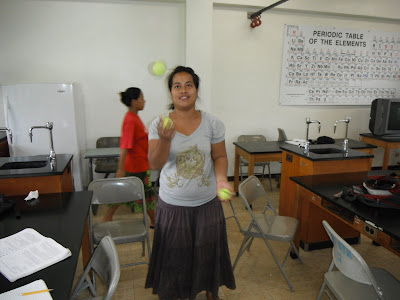Cuteleen

There are other mathematical relationships that govern physical systems. There are systems that are modeled by exponential and logarithmic functions. The path of a RipStik formed a sine wave on a sheet of paper. There are exotic functions such as the hyperbolic sine and hyperbolic cosine. Some systems are best described by complex variables that include a real and an imaginary component. Many of these systems are beyond the mathematical scope of this course.

The relationships described above are algebraic mathematical models. Much of the mathematics curriculum is centered on algebra in part because algebra is important to describing the physical world. There are, however, other mathematical models, non-algebraic models. This laboratory seeks to broaden the students mathematical horizons by introducing a mathematical model and notation that is not algebraic. In laboratory fifteen the students were introduced to the mathematics of site swaps.Jeffrey does a little dance

In an attempt to connect site swap theory back to the language of algebraic equations, after introducing site swap notation I referred to sequences such as 33342333 as site swap equations. The sequence is a mathematical statement that can be true (juggable) or false (not juggable).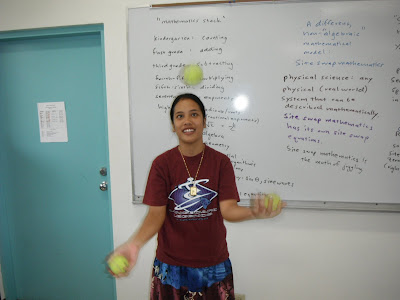This laboratory continues to provide a fun way to wrap up a term of exploring the mathematics at the core of physical science while expanding the students thinking with a mathematics system like nothing they have ever seen before.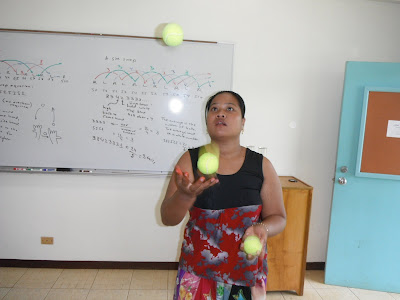Lizmay

The laboratory is also an end of term enjoyable experience. As the course is not required by any major, the students are primarily from majors other than those in the natural sciences. For many of these students science is a requirement, possibly even a dreaded requirement.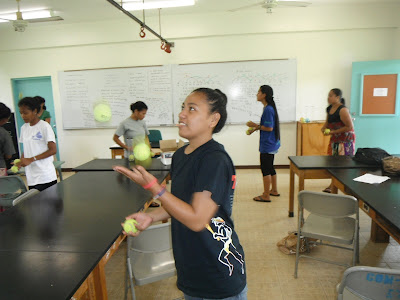Jessica Herry

I want the students to have the chance to do science, engage in exploring systems, grapple with the mathematical language underneath physical science. I can only hope that the students catch a glimpse of the beauty of science - of even pure science for science's sake.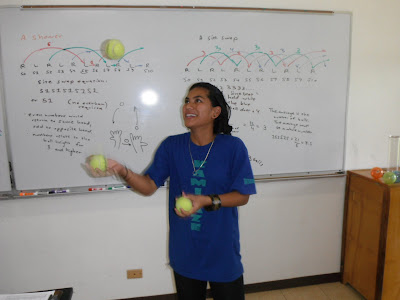Roxanne

I want the students to have good memories of the course, to think positively of science and of their own ability to do science. Thus I try to end the course on George M. Cohan's advice to "Always leave them laughing when you say goodbye."Tracy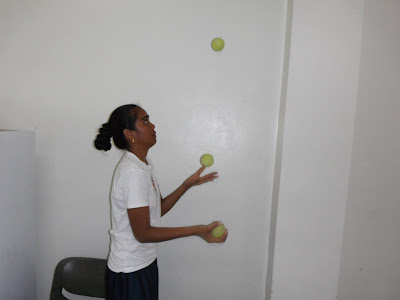HannaBeverlyn